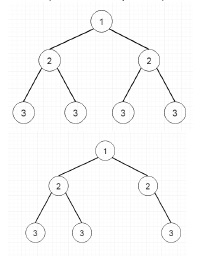# Symmetric Tree in Python

PythonServer Side ProgrammingProgramming

Suppose we have one binary tree. We have to check whether the tree is a symmetric tree or not. A tree will be said to be symmetric if it is the same when we take the mirror image of it. From these two trees, the first one is symmetric, but the second one is not.To solve this, we will follow these steps.

• We will call following steps recursively. The function will be solve(root, root)
• if the node1 and node2 are empty, then return true
• if either node1 or node2 is empty, then return false
• return true when node1.val = node2.val and solve(node1.left, node2.right) and solve(node1.right, node2.left)

Let us see the following implementation to get better understanding −

## Example

Live Demo

class TreeNode:
def __init__(self, data, left = None, right = None):
self.data = data
self.left = left
self.right = right
def insert(temp,data):
que = []
que.append(temp)
while (len(que)):
temp = que
que.pop(0)
if (not temp.left):
if data is not None:
temp.left = TreeNode(data)
else:
temp.left = TreeNode(0)
break
else:
que.append(temp.left)
if (not temp.right):
if data is not None:
temp.right = TreeNode(data)
else:
temp.right = TreeNode(0)
break
else:
que.append(temp.right)
def make_tree(elements):
Tree = TreeNode(elements)
for element in elements[1:]:
insert(Tree, element)
return Tree
class Solution(object):
def isSymmetric(self, root):
"""
:type root: TreeNode
:rtype: bool
"""
return self.solve(root,root)
def solve(self,node1,node2):
if not node1 and not node2:
return True
if not node1 or not node2:
return False
# print(node1.val, node2.val)
return node1.data == node2.data and
self.solve(node1.left,node2.right) and
self.solve(node1.right,node2.left)
tree1 = make_tree([1,2,2,3,4,4,3])
tree2 = make_tree([1,2,2,3,4,None,3])
ob1 = Solution()
print(ob1.isSymmetric(tree1))
print(ob1.isSymmetric(tree2))

## Input

tree1 = make_tree([1,2,2,3,4,4,3])
tree2 = make_tree([1,2,2,3,4,None,3])

## Output

True
False